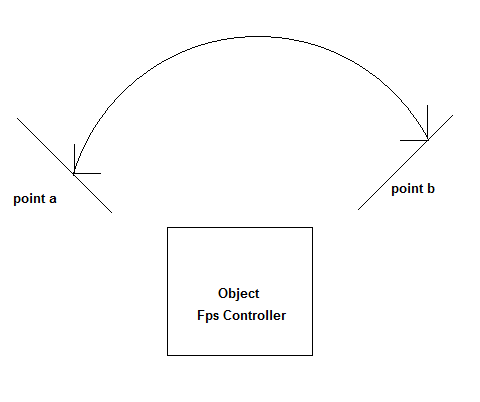# FPS Controller rotate between two points c#

Hello
I cant seem to rotate an object “FPS Controller” between two points. The below image will describe what I meanI want to rotate the fps contoller between those two points instead of the normal 360 degree rotation, going back and forth between them. I am using c# scripts to do everything else in the game.

Any help would be appreciated

You could try to use a script like this in your Update()

(C#)

``````Vector3 origin, goal; //euler angles
float speed;

void Update()
{
transform.rotation = Quaternion.Euler(Vector3.LerpAngle(origin, goal, Mathf.PingPong(Time.time * speed, 1.0f)));
}
``````

using UnityEngine;
using System.Collections;

``````public class rotateCharacter : MonoBehaviour {
public float minAngle = 50.0f;
public float maxAngle = 130.0f;
public float speed = 2.5f;

// Use this for initialization
void Start () {

}

// Update is called once per frame
void Update () {
float angle = Mathf.LerpAngle(minAngle, maxAngle, Mathf.PingPong(Time.time / speed, 1.0f));
transform.eulerAngles = new Vector3(0, angle, 0);
}
``````

}

this works perfectly

Thanks for everyones input and help